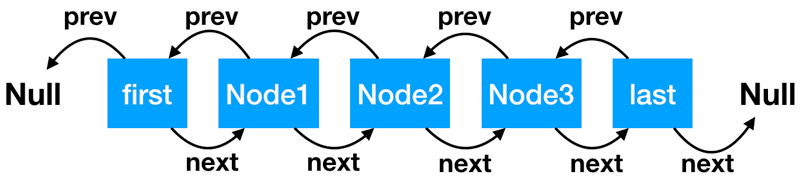• Node: 代表链中的每个节，Node 的 prev 属性，代表前一个节点的地址，Node 的next 属性，代表后一个节点的地址；
• first :代表双向链表的头节点，它的前一个节点是 null。
• last: 代表双向链表的尾节点，它的后一个节点是 null；
• 如果链表中没有任何数据时，头节点first 和 尾节点last 是同一个节点，前后指向都是 null；

``private static class Node<E> {``    E item;// 节点中存储的数据``    Node<E> next; // 下一个节点的地址``    Node<E> prev; // 前一个节点的地址``    // 构造方法初始化参数顺序分别是：前一个节点的地址值、当前节点中存储的数据、后一个节点的地址值``    Node(Node<E> prev, E element, Node<E> next) {``        this.item = element;``        this.next = next;``        this.prev = prev;``    }``}``

### 2.1 添加（新增）节点

``// 从尾部开始添加节点``void linkLast(E e) {``    // 把尾节点数据暂存``    final Node<E> l = last;``    // 新建新的节点，初始化入参含义：``    // l 是新节点的前一个节点，当前值是尾节点值``    // e 表示当前新增节点，当前新增节点后一个节点是 null``    final Node<E> newNode = new Node<>(l, e, null);``    // 新建节点添加到尾部``    last = newNode;``    //如果链表为空（l 是尾节点，尾节点为空，链表即空），头部和尾部是同一个节点，都是新建的节点``    if (l == null)``        first = newNode;``        //否则把前尾节点的下一个节点，指向当前尾节点。``    else``        l.next = newNode;    ``    size++;//集合元素数量增加1``    modCount++;//实际修改次数增加1``}``

``// 从头部添加``private void linkFirst(E e) {``    // 头节点赋值给临时变量``    final Node<E> f = first;``    // 新建节点，前一个节点指向null，e 是新建节点，f 是新建节点的下一个节点，目前值是头节点的值``    final Node<E> newNode = new Node<>(null, e, f);``    // 新建节点成为头节点``    first = newNode;``    // 头节点为空，就是链表为空，头尾节点是一个节点``    if (f == null)``        last = newNode;``    //上一个头节点的前一个节点指向当前节点``    else``        f.prev = newNode;``    size++;``    modCount++;``}``

### 2.2 删除节点

``//从头删除节点 f 是链表头节点``private E unlinkFirst(Node<E> f) {``    // 拿出头节点的值，作为方法的返回值``    final E element = f.item;``    // 拿出头节点的下一个节点``    final Node<E> next = f.next;``    //帮助 GC 回收头节点``    f.item = null;``    f.next = null;``    // 头节点的下一个节点成为头节点``    first = next;``    //如果 next 为空，表明链表为空``    if (next == null)``        last = null;``    //链表不为空，头节点的前一个节点指向 null``    else``        next.prev = null;``    //修改链表大小和版本``    size--;``    modCount++;``    return element;``}``

### 2.3 查询节点

``// 根据链表索引位置查询节点``Node<E> node(int index) {``    // 如果 index 处于队列的前半部分，从头开始找，size >> 1 是 size 除以 2 的意思。``    if (index < (size >> 1)) {``        Node<E> x = first;``        // 直到 for 循环到 index 的前一个 node 停止``        for (int i = 0; i < index; i++)``            x = x.next;``        return x;``    } else {// 如果 index 处于队列的后半部分，从尾开始找``        Node<E> x = last;``        // 直到 for 循环到 index 的后一个 node 停止``        for (int i = size - 1; i > index; i--)``            x = x.prev;``        return x;``    }``}``

### 2.4 迭代器

``// 双向迭代器``private class ListItr implements ListIterator<E> {``    private Node<E> lastReturned;//上一次执行 next() 或者 previos() 方法时的节点位置``    private Node<E> next;//下一个节点``    private int nextIndex;//下一个节点的位置``    //expectedModCount：期望版本号；modCount：目前最新版本号``    private int expectedModCount = modCount;``    …………``}``

``// 判断还有没有下一个元素``public boolean hasNext() {``    return nextIndex < size;// 下一个节点的索引小于链表的大小，就有``}``// 取下一个元素``public E next() {``    //检查期望版本号有无发生变化``    checkForComodification();``    if (!hasNext())//再次检查``        throw new NoSuchElementException();``    // next 是当前节点，在上一次执行 next() 方法时被赋值的。``    // 第一次执行时，是在初始化迭代器的时候，next 被赋值的``    lastReturned = next;``    // next 是下一个节点了，为下次迭代做准备``    next = next.next;``    nextIndex++;``    return lastReturned.item;``}``

``// 如果上次节点索引位置大于 0，就还有节点可以迭代``public boolean hasPrevious() {``    return nextIndex > 0;``}``// 取前一个节点``public E previous() {``    checkForComodification();``    if (!hasPrevious())``        throw new NoSuchElementException();``    // next 为空场景：1:说明是第一次迭代，取尾节点(last);2:上一次操作把尾节点删除掉了``    // next 不为空场景：说明已经发生过迭代了，直接取前一个节点即可(next.prev)``    lastReturned = next = (next == null) ? last : next.prev;``    // 索引位置变化``    nextIndex--;``    return lastReturned.item;``}``

``public void remove() {``    checkForComodification();``    // lastReturned 是本次迭代需要删除的值，分以下空和非空两种情况：``    // lastReturned 为空，说明调用者没有主动执行过 next() 或者 previos()，直接报错``    // lastReturned 不为空，是在上次执行 next() 或者 previos()方法时赋的值``    if (lastReturned == null)``        throw new IllegalStateException();``    Node<E> lastNext = lastReturned.next;``    //删除当前节点``    unlink(lastReturned);``    // next == lastReturned 的场景分析：从尾到头递归顺序，并且是第一次迭代，并且要删除最后一个元素的情况下``    // 这种情况下，previous() 方法里面设置了 lastReturned = next = last,所以 next 和 lastReturned会相等``    if (next == lastReturned)``        // 这时候 lastReturned 是尾节点，lastNext 是 null，所以 next 也是 null，这样在 previous() 执行时，发现 next 是 null，就会把尾节点赋值给 next``        next = lastNext;``    else``        nextIndex--;``    lastReturned = null;``    expectedModCount++;``}``